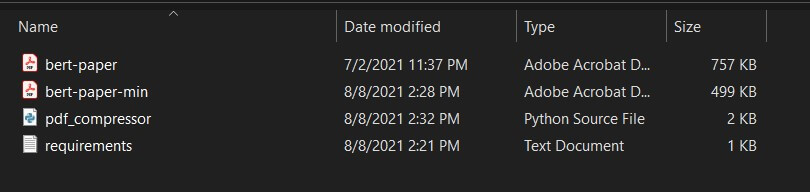# How to Compress PDF Files in Python

Learn how to compress PDF files in Python using the wrapper of PDFTron SDK.
4 min read · Updated feb 2022 · PDF File Handling

Compressing PDF allows you to decrease the file size as small as possible while maintaining the quality of the media in that PDF file. As a result, it significantly increases effectiveness and shareability.

In this tutorial, you will learn how to compress PDF files using the PDFTron library in Python.

PDFNetPython3 is a wrapper for PDFTron SDK. With PDFTron components, you can build reliable & speedy applications that can view, create, print, edit, and annotate PDFs across various operating systems. Developers use PDFTron SDK to read, write, and edit PDF documents compatible with all published versions of PDF specifications (including the latest ISO32000).

PDFTron is not freeware. It offers two types of licenses depending on whether you're developing an external/commercial product or an in-house solution.

We will use the free trial version of this SDK for this tutorial. The goal of this tutorial is to develop a lightweight command-line-based utility through Python-based modules without relying on external utilities outside the Python ecosystem (e.g., Ghostscript) that compress PDF files.

Note that this tutorial only works for compressing PDF files and not any file. You can check this tutorial for compressing and archiving files.

Read also: How to Compress Images in Python.

To get started, let's install the Python wrapper using pip:

``\$ pip install PDFNetPython3==8.1.0``

Open up a new Python file and import necessary modules:

``````# Import Libraries
import os
import sys
from PDFNetPython3.PDFNetPython import PDFDoc, Optimizer, SDFDoc, PDFNet``````

Next, let's define a function that prints the file size in the appropriate format (grabbed from this tutorial):

``````def get_size_format(b, factor=1024, suffix="B"):
"""
Scale bytes to its proper byte format
e.g:
1253656 => '1.20MB'
1253656678 => '1.17GB'
"""
for unit in ["", "K", "M", "G", "T", "P", "E", "Z"]:
if b < factor:
return f"{b:.2f}{unit}{suffix}"
b /= factor
return f"{b:.2f}Y{suffix}"``````

Now let's define our core function:

``````def compress_file(input_file: str, output_file: str):
"""Compress PDF file"""
if not output_file:
output_file = input_file
initial_size = os.path.getsize(input_file)
try:
# Initialize the library
PDFNet.Initialize()
doc = PDFDoc(input_file)
# Optimize PDF with the default settings
doc.InitSecurityHandler()
# Reduce PDF size by removing redundant information and compressing data streams
Optimizer.Optimize(doc)
doc.Save(output_file, SDFDoc.e_linearized)
doc.Close()
except Exception as e:
print("Error compress_file=", e)
doc.Close()
return False
compressed_size = os.path.getsize(output_file)
ratio = 1 - (compressed_size / initial_size)
summary = {
"Input File": input_file, "Initial Size": get_size_format(initial_size),
"Output File": output_file, f"Compressed Size": get_size_format(compressed_size),
"Compression Ratio": "{0:.3%}.".format(ratio)
}
# Printing Summary
print("## Summary ########################################################")
print("\n".join("{}:{}".format(i, j) for i, j in summary.items()))
print("###################################################################")
return True``````

This function compresses a PDF file by removing redundant information and compressing the data streams; it then prints a summary showing the compression ratio and the size of the file after compression. It takes the PDF `input_file` and produces the compressed PDF `output_file`.

Now let's define our main code:

``````if __name__ == "__main__":
# Parsing command line arguments entered by user
input_file = sys.argv
output_file = sys.argv
compress_file(input_file, output_file)``````

We simply get the input and output files from the command-line arguments and then use our defined `compress_file()` function to compress the PDF file.

Let's test it out:

``\$ python pdf_compressor.py bert-paper.pdf bert-paper-min.pdf``

The following is the output:

``````PDFNet is running in demo mode.
Permission: optimizer
Permission: write
## Summary ########################################################
Input File:bert-paper.pdf
Initial Size:757.00KB
Output File:bert-paper-min.pdf
Compressed Size:498.33KB
Compression Ratio:34.171%.
###################################################################``````

As you can see, a new compressed PDF file with the size of `498KB` instead of `757KB`. Check this out:

##Conclusion

Here are some other related PDF tutorials:

Check the complete code here.

Happy coding ♥

Sharing is caring!